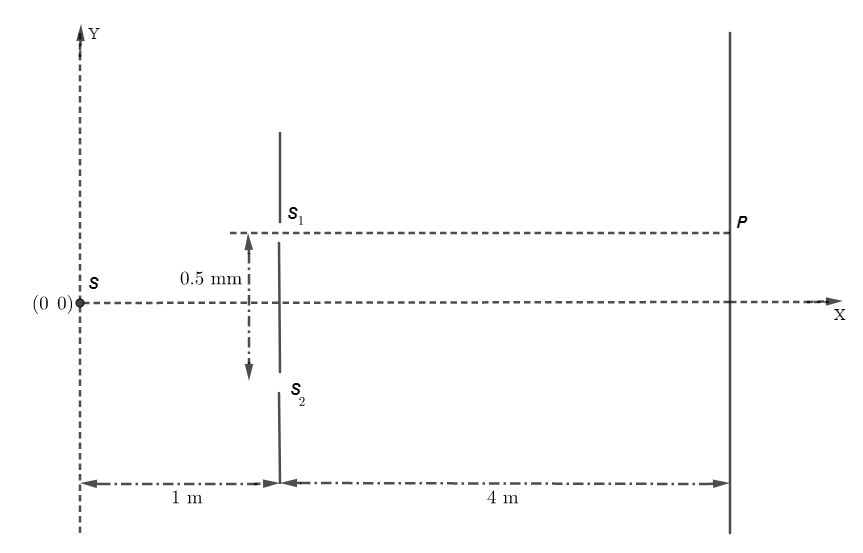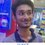# Please help with this YDSE problem.The figure shows a YDSE set-up. The source S of wavelength $\lambda = 4000 A^{\circ}$ oscillates along the y-axis according to the equation $y = \sin(\pi t)$ where $y$ is in millimetres and $t$ is in seconds. The distance between the two slits is $0.5 \text{ mm}$. Answer the following questions:

Q. The instant at which maximum intensity occurs at $P$ for the first time is:

• $\dfrac{1}{\pi} \arcsin \left( \dfrac{59}{160} \right)$

• $\dfrac{1}{\pi} \arcsin \left( \dfrac{59}{80} \right)$

• $\dfrac{1}{\pi} \arcsin \left( \dfrac{27}{160} \right)$

• $\dfrac{1}{\pi} \arcsin \left( \dfrac{27}{80} \right)$

Q. The instant at which minimum intensity occurs at $P$ for the first time is:

• $\dfrac{1}{\pi} \arcsin \left( \dfrac{59}{160} \right)$

• $\dfrac{1}{\pi} \arcsin \left( \dfrac{59}{80} \right)$

• $\dfrac{1}{\pi} \arcsin \left( \dfrac{27}{160} \right)$

• $\dfrac{1}{\pi} \arcsin \left( \dfrac{27}{80} \right)$Note by Tapas Mazumdar
3 years, 1 month ago

This discussion board is a place to discuss our Daily Challenges and the math and science related to those challenges. Explanations are more than just a solution — they should explain the steps and thinking strategies that you used to obtain the solution. Comments should further the discussion of math and science.

When posting on Brilliant:

• Use the emojis to react to an explanation, whether you're congratulating a job well done , or just really confused .
• Ask specific questions about the challenge or the steps in somebody's explanation. Well-posed questions can add a lot to the discussion, but posting "I don't understand!" doesn't help anyone.
• Try to contribute something new to the discussion, whether it is an extension, generalization or other idea related to the challenge.
• Stay on topic — we're all here to learn more about math and science, not to hear about your favorite get-rich-quick scheme or current world events.

MarkdownAppears as
*italics* or _italics_ italics
**bold** or __bold__ bold
- bulleted- list
• bulleted
• list
1. numbered2. list
1. numbered
2. list
Note: you must add a full line of space before and after lists for them to show up correctly
paragraph 1paragraph 2

paragraph 1

paragraph 2

[example link](https://brilliant.org)example link
> This is a quote
This is a quote
    # I indented these lines
# 4 spaces, and now they show
# up as a code block.

print "hello world"
# I indented these lines
# 4 spaces, and now they show
# up as a code block.

print "hello world"
MathAppears as
Remember to wrap math in $$ ... $$ or $ ... $ to ensure proper formatting.
2 \times 3 $2 \times 3$
2^{34} $2^{34}$
a_{i-1} $a_{i-1}$
\frac{2}{3} $\frac{2}{3}$
\sqrt{2} $\sqrt{2}$
\sum_{i=1}^3 $\sum_{i=1}^3$
\sin \theta $\sin \theta$
\boxed{123} $\boxed{123}$

## Comments

Sort by:

Top Newest

@Steven Chase @Mark Hennings @Md Zuhair Please help.

- 3 years, 1 month ago

Log in to reply

Question 1- (B)?

- 3 years, 1 month ago

Log in to reply

Question 2-(D)?

- 3 years, 1 month ago

Log in to reply

@Sahil Silare can u write the solution

- 3 years, 1 month ago

Log in to reply

I'm bad with latex but what I did was adding up the path difference from source to slits and slits to screen and then it will be equal to n(lambda) for maximum.

- 3 years, 1 month ago

Log in to reply

×

Problem Loading...

Note Loading...

Set Loading...